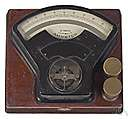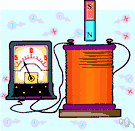# ampere

(redirected from Andre-Marie Ampere)
Also found in: Thesaurus, Medical, Encyclopedia.
Related to Andre-Marie Ampere: Georg Simon Ohm, Michael Faraday, Alessandro Volta

## am·pere

(ăm′pîr′)
n. Abbr. A
The basic unit of electric current, equal to one coulomb per second and equivalent to the current, flowing in two straight parallel wires of negligible cross section separated by a distance of one meter, that produces a force between the wires of 2.0 × 10-7 newtons per meter of length. The value of an ampere in the International System differs very slightly from that in the meter-kilogram-second-ampere system of units. See Table at measurement.

[After André Marie Ampère.]

## Ampère

(ˈæmpɛə; French ɑ̃pɛr)
n
(Biography) André Marie (ɑ̃dre mari). 1775–1836, French physicist and mathematician, who made major discoveries in the fields of magnetism and electricity

## ampere

(ˈæmpɛə)
n
1. (Units) the basic SI unit of electric current; the constant current that, when maintained in two parallel conductors of infinite length and negligible cross section placed 1 metre apart in free space, produces a force of 2 × 10–7 newton per metre between them. 1 ampere is equivalent to 1 coulomb per second
2. (Units) a former unit of electric current (international ampere); the current that, when passed through a solution of silver nitrate, deposits silver at the rate of 0.001118 gram per second. 1 international ampere equals 0.999835 ampere
Abbreviation: amp Symbol: A
[C19: named after André Marie Ampère]

## am•pere

(ˈæm pɪər, æmˈpɪər)

n.
the SI unit of electrical current, equal to a constant current that would produce a force of 2 x 10−7 newton per meter of length when maintained in two straight parallel conductors of infinite length and negligible circular cross section and placed one meter apart in a vacuum. Abbr.: A, amp.
[1881; after A. M. Ampère]

## Am•père

(ˈæm pɪər, æmˈpɪər, Fr. ɑ̃ˈpɛr)

n.
André Marie, 1775–1836, French physicist.

## am·pere

(ăm′pîr′)
A unit used to measure electric current. Electric current is measured by how great a charge passes a given point in a second. One ampere is equal to a flow of one coulomb per second.

## ampere

1. The unit of electric current in the international system.
2. (A) The unit for measuring electric current.
ThesaurusAntonymsRelated WordsSynonymsLegend:
 Noun 1ampere - a former unit of electric current (slightly smaller than the SI ampere)international amperecurrent unit - a measure of the amount of electric charge flowing past a circuit point at a specific time 2ampere - the basic unit of electric current adopted under the Systeme International d'Unites; "a typical household circuit carries 15 to 50 amps"amp, Acurrent unit - a measure of the amount of electric charge flowing past a circuit point at a specific timemilliampere, mA - one thousandth of an ampereabamp, abampere - a unit of current equal to 10 amperes
Translations
ampér
ampere
amper
ampeeri
amper
amper
amper
アンペア
amper
ampér
amper
ampere

## ampere

ampère [ˈæmpɛəʳ]
A. N
B. CPD ampere-hour Namperio-hora m

## ampere

ampère [ˈæmpɛər] (British) n

## ampère

(ˈӕmpeə) noun
(also amp (ӕmp) ) (often abbreviated to A when written) the unit by which an electric current is measured.
References in periodicals archive ?
The French physicist Andre-Marie Ampere used the term 'cybernetique' in his 1834 essay "Essai sur la philosophie des sciences", again in reference to the sciences of government.
Tresch details how Andre-Marie Ampere, together with Arago, built on Oersted's findings, deducing the basic principles of electrodynamics in the process.

Site: Follow: Share:
Open / Close